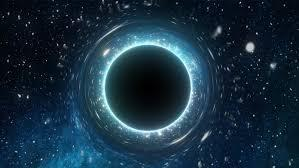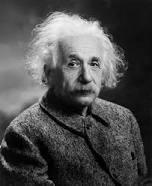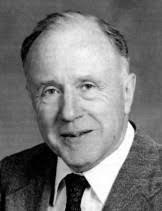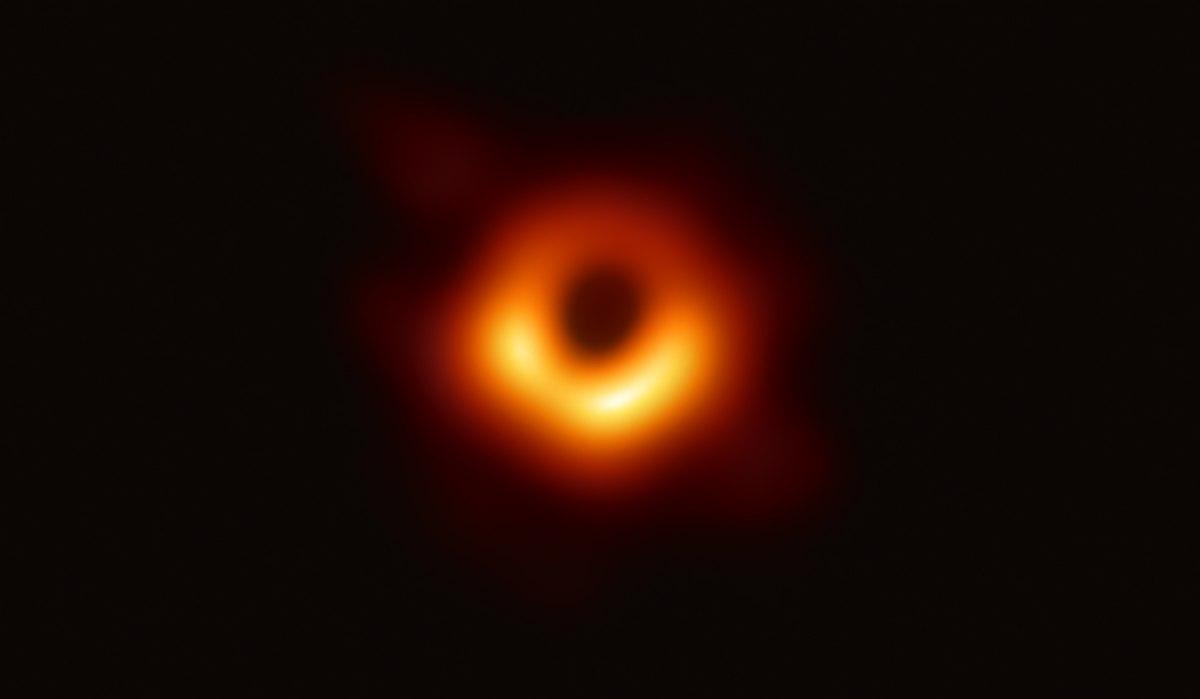# BLACK HOLES

In the year 1916, Albert Einstein predicted the existence of Black Holes as a region in spacetime where the gravitational force of an object is so much that not even light can escape from there. The term “Black Hole” was coined by American astronomer John Wheeler many years later in 1967.Black HoleAlbert Einstein    John Wheeler

The theory of General Relativity predicted that any sufficiently compact mass can deform spacetime to form a black hole. The point of no return from which nothing can return after falling under the gravitational pull of a black hole is known as event horizon. As important as the significance of the event horizon is in a black hole, it has no detectable features as predicted by the General Theory of Relativity.

Black Holes emit no light; therefore, they act like an ideal Black Hole. However as strange as it may sound, Black Holes are not eternal. Quantum field theory in curved space time predicts that event horizon of a black hole emits Hawking Radiation with a temperature inversely proportional to its mass and spectrum similar to that of a black body. However, the temperature is of the order of billionths of kelvin of Black Holes, therefore making it nearly impossible to observe it from Earth.

The first solution to the Einstein field equations of General Theory of relativity was given by Karl Schwarzschild in 1916 known Schwarzschild Radius (SR). This gives the formulae relating to the radius corresponding to the event horizon of a Black Hole and the Gravitational Constant (G), mass of the black hole (M) and speed of light. It is given by:

rs=2GMc2

How they are formed?

Black Holes are expected to be formed when compact stars (with a radius smaller than it’s Schwarzschild radius) at the end of their life cycle undergo gravitational collapse when the inward gravitational pull overcomes the outward nuclear force. A black hole by definition does not occupy any space but has the mass equivalent to the mass of the star compressed to a single point.

Classification of Black Hole by Schwarzschild Radius

Any object with a radius smaller than its Schwarzschild radius is defined as a Black Hole. The surface of a non-rotating black hole at the Schwarzschild radius acts as an event horizon. They can be equally classified on the basis of their density where density is defined as the mass of a black hole divided by the volume of its Schwarzschild radius. Volume contained in the event horizon of the massive black hole has an average density lower than those of main sequence stars.

Black holes are further classified here as-

1. Supermassive black hole

Supermassive black holes are of the genre of the most massive black holes, with the masses of the order of hundred thousand to billions solar masses. For e.g.  Supermassive black hole NGC 4889 with a mass of 21 billion has been detected.

Assuming the given object has a constant mass-density, the SR is directly related to that mass which in turn is related to the volume. This implies that the physical radius of the body is directly proportional to the cube root of its volume. Therefore, as a given black hole will suck more mass from its surrounding, its SR will increase at a greater rate than the corresponding Physical radius.Supermassive black hole in galaxy M87 with a mass of 7 billion suns

1. Stellar Black Hole

Stellar mass black hole has comparatively greater average density than its supermassive counterpart. If we can accumulate a given amount of matter at nuclear density, then such an object would fall under its own Schwarzschild radius and therefore, we will get a stellar black hole.

1. Primordial Black Hole

Primordial black holes have masses equivalent to the size of a Mount Everest, such that its Schwarzschild radius will be less than a nanometer. The average density of such an object would be so high and dense that no known mechanism could produce that much energy in order to form such extremely compact objects. The formation of these Black holes was only possible at the time of Big Bang where densities of the corresponding medium were extremely high.

Written by- Sukhjit Singh

He is a bachelor student at SGTB Khalsa College pursuing B.Sc. Mathematics honors third year. He often takes multiple exciting and aims to develop multiple skills from the same.

Edited by- Shweta Mittal, Team SciComm